Need Help?

Subscribe to Dynamic

###### \${selected_topic_name}
• Notes
• Comments & Questions

$\begin{array}{l}{\text { 17-2. The solid cylinder has an outer radius } R \text { , height } h \text { . }} \\ {\text { and is mado from a material having a clonsity that varics }}\end{array}$

$\begin{array}{l}{\text { From its conter as } \rho=k+\text { ar }^{2} \text { . Where } k \text { and } a \text { are constants. }} \\ {\text { Determine the mass of the eylinder and its moment of }} \\ {\text { inertia about the } z \text { ax is. }}\end{array}$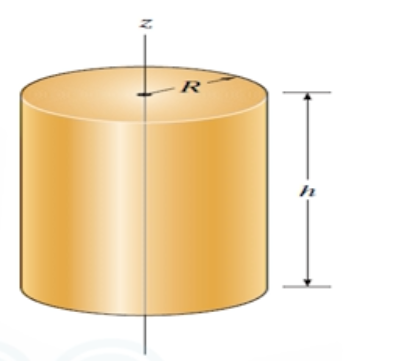$\rho=k+{a r}^{2}$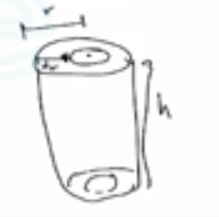$\mathrm{shell}$

$r \rightarrow d m$

$∵ dm=\rho d{v}=\rho(2 \pi r d r) h$

$∴ m=\int_{0}^{R}\left(k+a r^{2}\right)(2 \pi r d r) h$

$=2 \pi h \int_{0}^{R}\left(k r+a r^{3}\right) d r = 2 \pi h\left(\frac{k R^{2}}{2}+\frac{a k^{4}}{4}\right) \$

$m=\pi h R^{2}\left(k+\frac{a K^{2}}{2}\right)$

$∵ d I=r^{2} dm=r^{{2}} \rho(2 \pi r d r) h$

$∴I_{z}=\int_{0}^{R} r^{2}\left(k+a r^{2}\right)(2 \pi r d r) h=2 \pi h \int_{0}^{R}\left(k r^{3}+a r^{5}\right) d r$

$∴I_{z}=2 \pi h\left[\frac{k R^{4}}{4}+\frac{a R^{6}}{6}\right] \Rightarrow I_{z}= \frac{\pi h{R}^{4}}{2}\left[k+\frac{2 a R^{2}}{3}\right]$

$\begin{array}{l}{17-10 . \text { The pendulum consists of a } 4-\mathrm{kg} \text { circular plate and }} \\ {\text { a } 2-\mathrm{kg} \text { slender rod. Determine the radius of gyration of the }} \\ {\text { pendulum about an axis perpendicular to the page and }} \\ {\text { passing through point } \text{O} \text { . }}\end{array}$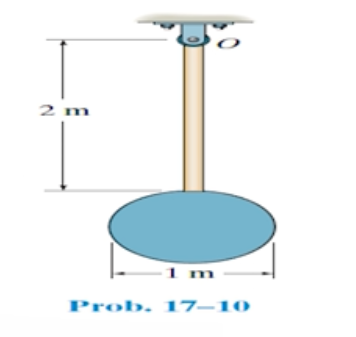$m_{c}=4 k g$

$m_{r}=2 k_{2}$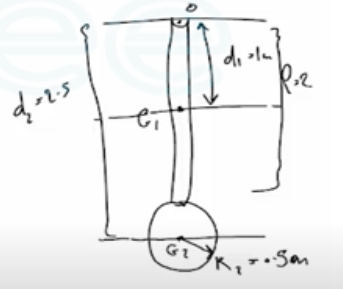$I_{0}=\Sigma(I_G+md^2$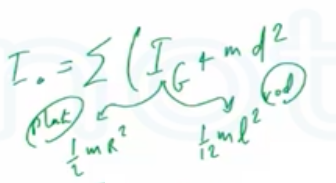$I_{0}=\left[\frac{1}{12} m l^{2}+m_{r} d_{1}^{2}\right]+\left[\frac{1}{2} ml^{2}+m_{c} d_{2}^{2}\right]$

$=\left[\frac{1}{12}(2)(2)^{2}+2(1)^{2}\right]+\left[\frac{1}{2}(4)(0.5)^{2}+4(2.5)^{2}\right]$

$=28.17 \mathrm{kg}.\mathrm{m}^{2}$

$k_{0}=\sqrt{\frac{I_0}{m_{t}}}=\sqrt{\frac{28.17}{4+2}}=2.167 \mathrm{m}=2.17m$

No comments yet